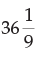Courses

# The sum of the series 24, 3, 8, 1, 2, 7,… to 8 terms isa)36b)54c)d)none of theseCorrect answer is option 'B'. Can you explain this answer? Related Test: Test: Arithmetic And Geometric Progressions - 1

## CA Foundation QuestionSNEHA VERMA Feb 19, 2020
Sum of series 24,3,8,1,2,7..to 8th term is:
Take the 1st and 2nd terms, 24,3, put them together, 243 = 3^5.
Take the 3rd and 4th terms, 8,1, put them together, 81 = 3^4.
Take the 5th and 6th terms, 2,7, put them together, 27 = 3^3.
Take the 7th and 8th terms, 0,9, put them together, 09 = 3^2.
Sum of 8 terms = 24+3+8+1+2+7+0+9 = 54

This discussion on The sum of the series 24, 3, 8, 1, 2, 7,… to 8 terms isa)36b)54c)d)none of theseCorrect answer is option 'B'. Can you explain this answer? is done on EduRev Study Group by CA Foundation Students. The Questions and Answers of The sum of the series 24, 3, 8, 1, 2, 7,… to 8 terms isa)36b)54c)d)none of theseCorrect answer is option 'B'. Can you explain this answer? are solved by group of students and teacher of CA Foundation, which is also the largest student community of CA Foundation. If the answer is not available please wait for a while and a community member will probably answer this soon. You can study other questions, MCQs, videos and tests for CA Foundation on EduRev and even discuss your questions like The sum of the series 24, 3, 8, 1, 2, 7,… to 8 terms isa)36b)54c)d)none of theseCorrect answer is option 'B'. Can you explain this answer? over here on EduRev! Apart from being the largest CA Foundation community, EduRev has the largest solved Question bank for CA Foundation.